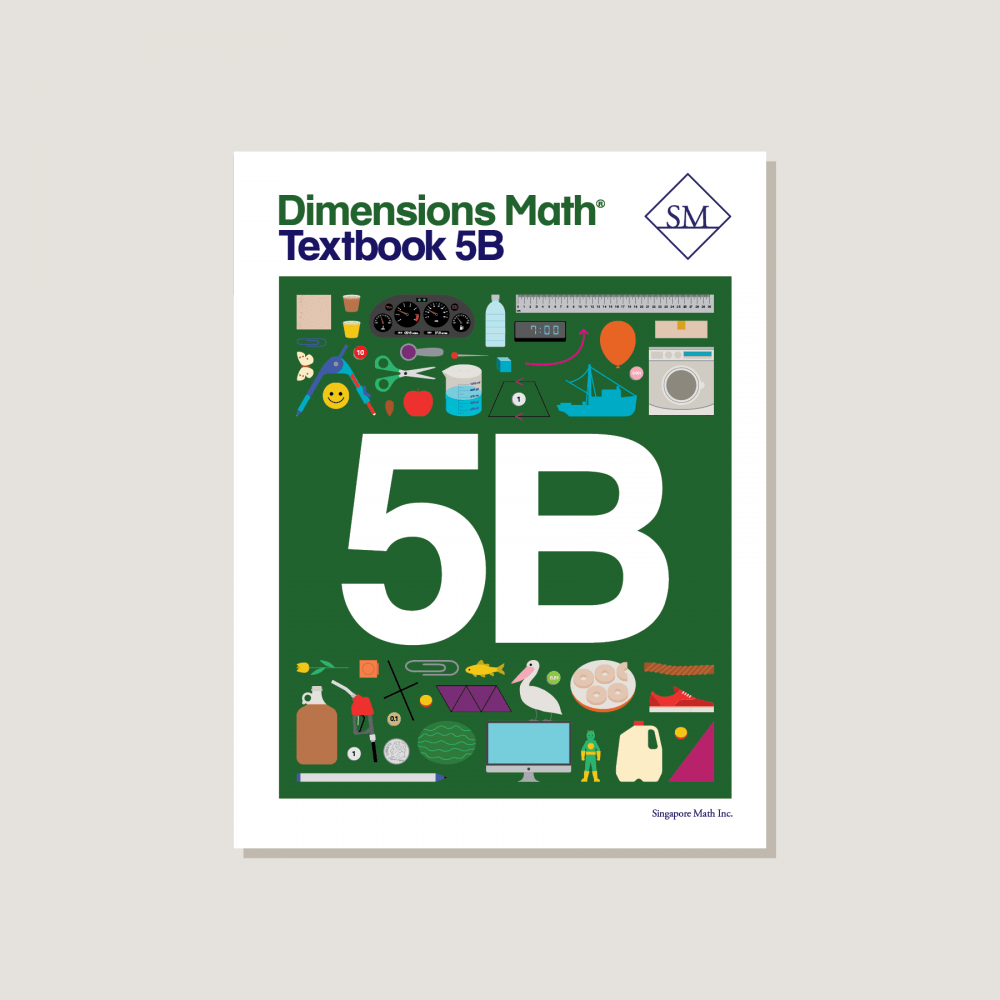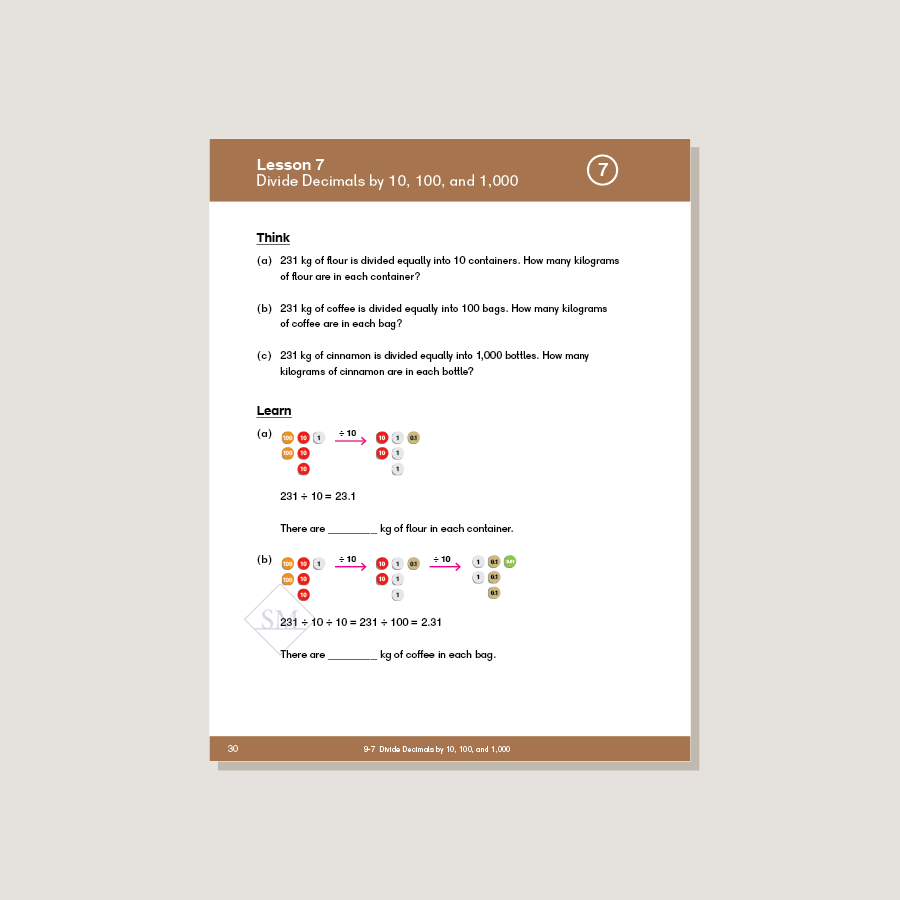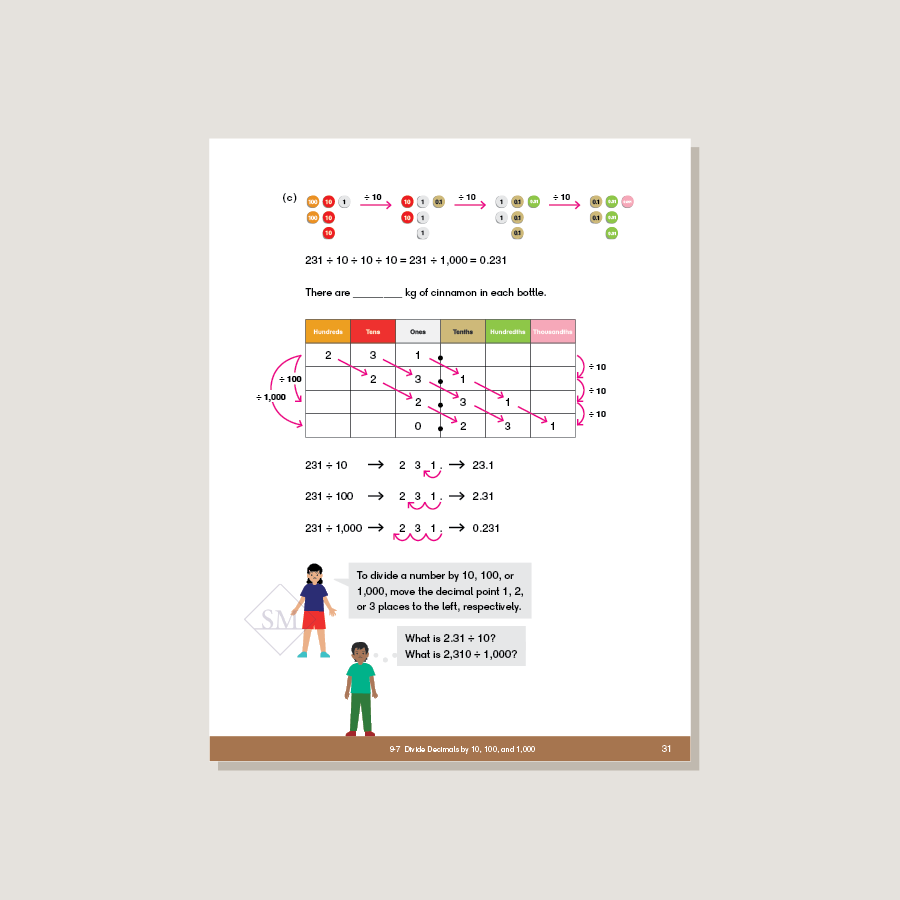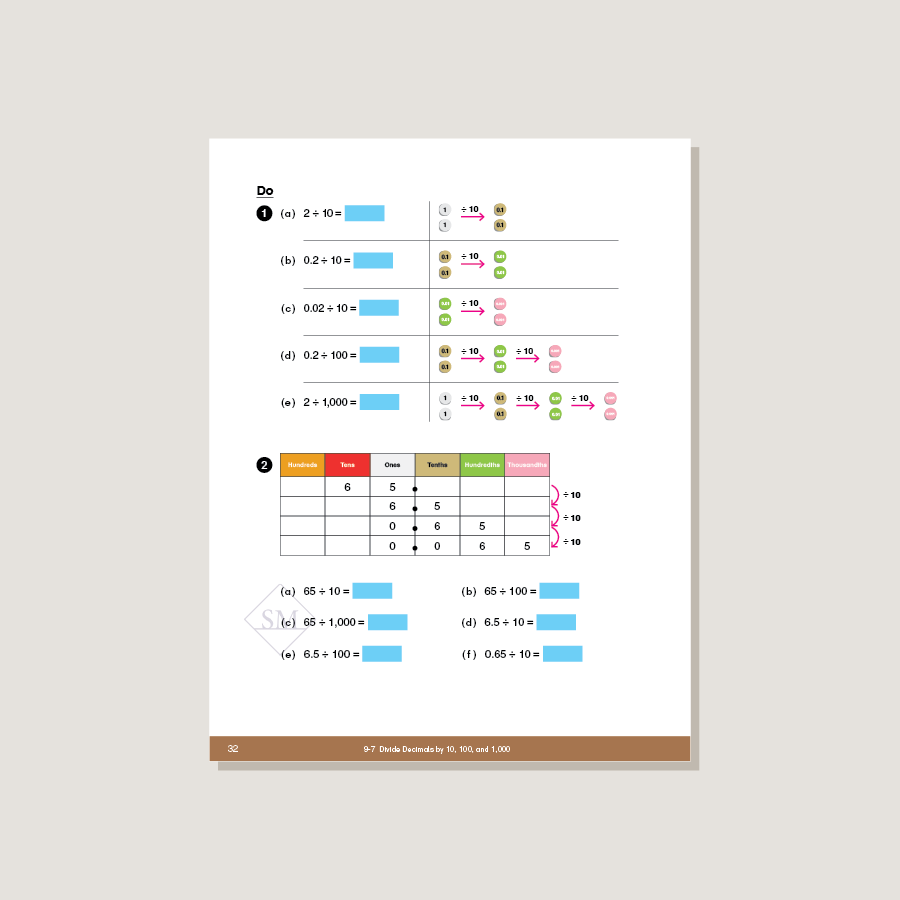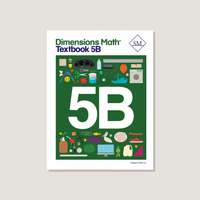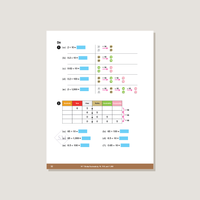# Dimensions Math Textbook 5B

\$12.80

Textbooks introduce concepts through logical sequencing. Lessons encourage discussion and help students reach fluency through engaging activities and strategies. Corresponding exercises in workbooks help students consolidate and practice new knowledge. Features & Components:
• Chapter Opener: Each chapter begins with an engaging scenario that stimulates student curiosity in new concepts. This scenario also provides teachers an opportunity to review skills.
• Think: With guidance from teachers, students solve a problem using a variety of methods.
• Learn: One or more solutions to the problem in Think are presented, along with definitions and other information to consolidate the concepts introduced in Think.
• Do: A variety of practice problems allow teachers to lead discussion or encourage independent mastery. These activities solidify and deepen student understanding of the concepts.
• Exercise: A pencil icon at the end of the lesson links to additional practice problems in the corresponding workbook.
• Practice: Periodic practice provides teachers with opportunities for consolidation, remediation, and assessment.
• Review: Two cumulative reviews provide ongoing practice of concepts and skills.
Note: Two textbooks (A and B) for each grade correspond to the two halves of the school year. Answer key not included. Soft cover.
SKU: DMT5B

ISBN: 9781947226135

Pagecount: 232

Dimensions: 8.5 x 11 x 0.75 in

Weight: 1 lbs

Binding: Standard

Color: Color

Cover: Soft

Perforated: No

Sample Pages

Chapter 9: Decimals
Lesson 1: Thousandths
Lesson 2: Place Value to Thousandths
Lesson 3: Comparing Decimals
Lesson 4: Rounding Decimals
Lesson 5: Practice A
Lesson 6: Multiply Decimals by 10, 100, and 1,000
Lesson 7: Divide Decimals by 10, 100, and 1,000
Lesson 8: Conversion of Measures
Lesson 9: Mental Calculation
Lesson 10: Practice B

Chapter 10: The Four Operations of Decimals
Lesson 1: Adding Decimals to Thousandths
Lesson 2: Subtracting Decimals
Lesson 3: Multiplying by 0.1 or 0.01
Lesson 4: Multiplying by a Decimal
Lesson 5: Practice A
Lesson 6: Dividing by a Whole Number — Part 1
Lesson 7: Dividing by a Whole Number — Part 2
Lesson 8: Dividing by a Whole Number by 0.1 and 0.01
Lesson 9: Dividing a Whole Number by a Decimal
Lesson 10: Practice B

Chapter 11: Geometry
Lesson 1: Measuring Angles
Lesson 2: Angles and Lines
Lesson 3: Classifying Triangles
Lesson 4: The Sum of the Angles in a Triangle
Lesson 5: The Exterior Angle of a Triangle
Lesson 6: Classifying Quadrilaterals
Lesson 7: Angles of Quadrilaterals — Part 1
Lesson 8: Angles of Quadrilaterals — Part 2
Lesson 9: Drawing Triangles and Quadrilaterals
Lesson 10: Practice

Chapter 12: Data Analysis and Graphs
Lesson 1: Average — Part 1
Lesson 2: Average — Part 2
Lesson 3: Line Plots
Lesson 4: Coordinate Graphs
Lesson 5: Straight Line Graphs
Lesson 6: Practice
Review 3

Chapter 13: RatioLesson 1: Finding the Ratio
Lesson 2: Equivalent Ratios
Lesson 3: Finding a Quantity
Lesson 4: Comparing Three Quantities
Lesson 5: Word Problems
Lesson 6: Practice

Chapter 14: Rate
Lesson 1: Finding the Rate
Lesson 2: Rate Problems — Part 1
Lesson 3: Rate Problems — Part 2
Lesson 4: Word Problems
Lesson 5: Practice

Chapter 15: Percentage
Lesson 1: Meaning of Percentage
Lesson 2: Expressing Percentages as Fractions
Lesson 3: Percentages and Decimals
Lesson 4: Expressing Fractions as Percentages
Lesson 5: Practice A
Lesson 6: Percentage of a Quantity
Lesson 7: Word Problems
Lesson 8: Practice B
Review 4
Review 5

A & B Books: Our programs divide the school year into two semesters. “A” level books are for the first half of the school year. “B” level books are for the second half of the school year. You need both “A” and “B” material for a complete school year.

Required Components: Textbooks, Workbooks, and Guides (either Home Instructor’s Guides or Teacher’s Guides) are all necessary components. These three elements each serve a unique function and work together to build math mastery.

Printouts: Dimensions Math PK-5 Resources

Recommended Manipulatives: Dimensions Math Grades PK-5 Recommended Manipulatives

Answer Key: Located in corresponding Teacher’s Guide

Dimensions Math At Home™ Videos

Invite a professional Singapore math teacher into your home classroom. This subscription of pre-recorded lessons covers all Textbook and Workbook material for an entire school year and can be done at your own pace.

Manipulatives

Manipulatives help students visualize and represent math concepts. Stock up on suggested items to deepen engagement during math activities and lessons.

Supplementary Math

Supplementary math helps students of all abilities: struggling, advanced, or on level. Find just the right addition to round out your core program.